Courses

# Test: Common Forces in Mechanics

## 20 Questions MCQ Test Physics Class 11 | Test: Common Forces in Mechanics

Description
This mock test of Test: Common Forces in Mechanics for JEE helps you for every JEE entrance exam. This contains 20 Multiple Choice Questions for JEE Test: Common Forces in Mechanics (mcq) to study with solutions a complete question bank. The solved questions answers in this Test: Common Forces in Mechanics quiz give you a good mix of easy questions and tough questions. JEE students definitely take this Test: Common Forces in Mechanics exercise for a better result in the exam. You can find other Test: Common Forces in Mechanics extra questions, long questions & short questions for JEE on EduRev as well by searching above.
QUESTION: 1

### A train is moving along a horizontal track. A pendulum suspended from the roof of the train makes an angle of 5° with the vertical. The acceleration of the train is

Solution:

Making the free body diagram of the pendulum bob we get
T.cosθ = mg
T.sinθ = ma (applying the concept of pseudo force)
By dividing the above two equations we get
tanθ = a/g
Thus we get a = g.tanθ
= 10 x tan 5°
= 0.87 ms-2

QUESTION: 2

### A body of weight 100 N is suspended with the help of strings as shown in figure.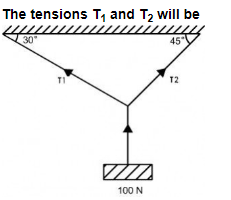Solution: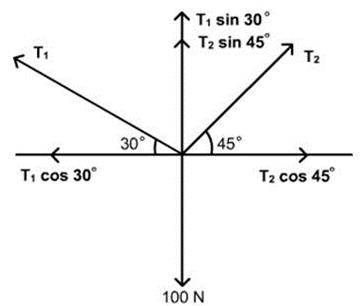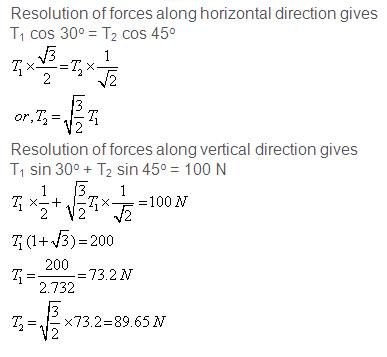QUESTION: 3

### For a body along the inclined plane, the normal reaction acts

Solution:

Normal force is the perpendicular component of the reaction force wrt the surface and thus it always acts perpendicularly to the surface.

QUESTION: 4

Two forces F1 and F2 are acting on a particle. The particle will remain at rest if two forces are

Solution:

For an object at rest to be at rest, no net acceleration must act upon it, which implies no net force. Thus the two forces need to nullify each other, which is only possible if both are equal but opposite.

QUESTION: 5

If no resultant force acts on a body then the body will be in

Solution:

What do you think about Newton's 1st law, he was said that " every body have nature to maintain inertia of rest or motion untill there is not net force applied on that body". means body will be earlier state (no change in state) when net force applied on that body equals zero.
I know, you thought answer is option (a). but this is not true. for better understanding, Let's take. an example. a body moves with uniform velocity then, net force applied on body = 0 because acceleration of body is zero . but here you see body is not in rest . it is in motion. it is in earlier state . its state doesn't change.

QUESTION: 6

A body is at rest though forces act on it. Then

Solution:

“Every body continues to be in its state of rest or of uniform motion in a straight line unless compelled by an unbalanced external force.” It is simply expressed as if the net external force on a body is zero, its acceleration is zero. Acceleration can be non-zero only if there is a net external force on the body.

QUESTION: 7

In mechanics, if the net external force on the particle is zero, then the particle is said to be in state of

Solution:

In mechanics, if the net external force on the particle is zero, then the particle is said to be in state of equilibrium. By definition.

QUESTION: 8

A book is lying on the table. What is the angle between the action of the book on the table and reaction of table on the book?

Solution:

The weight (W) and the action (A) both will act in same direction and parallel to each other. So the angle between them should be zero.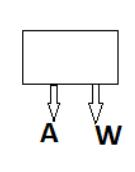QUESTION: 9

A mass of 6 kg is suspended by a rope of length 2 m from a ceiling. A force of 50 N in the horizontal direction is applied at the mid-point of the rope, as shown. What is the angle the rope makes with the vertical in equilibrium?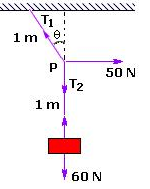Solution:

Making the free body diagram of the body we get
T1.cosθ = T2 = mg = 60
T1.sinθ = 50N
By dividing the above two equations we get
tanθ = 5/6
Thus we get θ = 40°

QUESTION: 10

Which of the conditions shows that the particles are in equilibrium state for two forces F1 and F2 acting on the body?

Solution:

At equilibrium condition net force on body is 0
F1 + F2 = 0
F1 = -F2

QUESTION: 11

Which of the following forces is not considered as a contact force in Mechanics?

Solution:

The force exerted by the earth on a body is called gravitational force. Actually this force exists between any two bodies in the universe.This force is always of attraction. e.g. When a body is dropped from a height it moves in downward direction towards the Earth with increasing speed (with constant acceleration). This constant acceleration by which all bodies fall down is called acceleration due to gravity. Its value is 9.8 m/s' (approx 10 m/s' )on the surface of the earth. e.g. i) A fruit from tree falls down;ii) Water falls down on a ground from a tap.iii) We feel the weight of bucket full of water holding in our hand.

QUESTION: 12

Weight and normal reaction forces

Solution: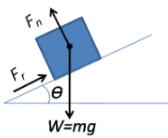In a simple case such as an object resting upon a table, the normal force on the object is equal but in opposite direction to the gravitational force applied on the object (or the weight of the object), that is, , where m is mass, and g is the gravitational field strength (about 9.81 m/s2 on Earth).

QUESTION: 13

The contact forces of mechanics are fundamentally derived from

Solution:

Friction is a surface phenomenon. When two surfaces have irregularities that come in contact do they get interlocked  preventing them against sliding each other. So, frictional force is a contact force dependent on the surface.
Gravitational force, electrostatic force and magnetic force does not depend on the surface.

QUESTION: 14

The minimum angle that an inclined plane makes with the horizontal when a body placed on it just begins to slide down is called

Solution:

The minimum angle that an inclined plane makes with the horizontal when a body placed on it just begins to slide down is called angle of repose.

QUESTION: 15

A body in equilibrium

Solution:

A body is said to be in equilibrium only when the net resultant of all external forces applied on the body becomes zero. It does not depend upon any of the internal forces because for any system internal forces exist in pairs because of Newton's third law  and hence they result in null.

QUESTION: 16

Spring force is a restoring force.

Solution:

The force exerted by a spring is called a restoring force; it always acts to restore the spring toward equilibrium. In Hooke's law, the negative sign on the spring's force means that the force exerted by the spring opposes the spring's displacement.

QUESTION: 17

The forces F1, F2, and F3 are acting on a particle of mass m, such that F2 and F3are mutually perpendicular and under the effect of F1, F2, and F3 , the particle remains stationary. What will be the acceleration of the particle, if the force F1 is removed?

Solution:

Concept: Forces.The particle is a stationary under the effect of forces F1, F2 and F3.
This shows that force F1 is equal and opposite to the resultant of forces F2 and F3.
Hence, if the force F1 is removed the particle will move under the action of the force -ve F1 and the acceleration will the particle will be,a =-F1/m.

QUESTION: 18

Tension on an almost massless rope being pulled by two boys, one small and one big, will be

Solution:

Because the Tension for rope at each point is always the same.

QUESTION: 19

Which of the following cannot be regarded as yet another kind of force?

Solution:

if an object is moving in a horizontal circle at constant speed, the centripetal force does not do any work and cannot alter the total mechanical energy of the object. For the reason, the kinetic energy and therefore the speed of the object will remain constant.

QUESTION: 20

The component of contact force normal to the surfaces in contact is called

Solution:

The component of contact force normal to the surfaces in contact is called normal reaction. The component parallel to the surfaces in contact is called friction.